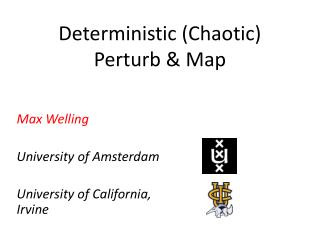# Deterministic (Chaotic) Perturb & Map - PowerPoint PPT PresentationDownload PresentationDeterministic (Chaotic) Perturb & Map

Deterministic (Chaotic) Perturb & MapDownload Presentation## Deterministic (Chaotic) Perturb & Map

- - - - - - - - - - - - - - - - - - - - - - - - - - - E N D - - - - - - - - - - - - - - - - - - - - - - - - - - -
##### Presentation Transcript

1. Deterministic (Chaotic)Perturb & Map Max Welling University of Amsterdam University of California, Irvine

2. Overview • Introduction herding though joint image segmentation and labelling. • Comparison herding and “Perturb and Map”. • Applications of both methods • Conclusions

3. Step I: Learn Good Classifiers • A classifier : images features X  object label y. • Image features are collected in square window around target pixel.

4. Step II: Use Edge Information • Probability : image features /edges  pairs of object labels. • For every pair of pixels compute the probability that they cross an object boundary.

5. Step III: Combine Information How do we combine classifier input and edge information into a segmentation algorithm? We will run a nonlinear dynamical system to sample many possible segmentations The average will be out final result.

6. The Herding Equations (y takes values {0,1} here for simplicity) average

7. Some Results local classifiers ground truth MRF herding

8. Dynamical System • The map represents a weakly chaotic nonlinear dynamical system. y=1 y=6 y=2 y=5 y=3 Itinerary: y=[1,1,2,5,2,… y=4

9. Geometric Interpretation

10. Convergence Translation: ChooseStsuchthat: Then: s=1 s=6 Equivalent to “Perceptron Cycling Theorem” (Minsky ’68) s=[1,1,2,5,2... s=2 s=5 s=3 s=4

11. Perturb and MAP Papandreou & Yuille, ICCV - 11 -Learn offset: using moment matching -Use Gumbel PDFs To add noise State: s2 State: s3 State: s1 State: s4 State: s6 State: s5

12. PaM vs. Frequentism vs. Bayes Given some likelihood P(x|w), how can you determine a predictive distribution P(x|X)? Given dataset X, and sampling-distr. P(Z|X), a bagging frequentist will: Sample fake data-set Z_t ~ P(Z|X) (e.g. by bootstrap sampling) Solve w*_t = argmax_w P(Z_t|w) Prediction P(x|X) ~ sum_t P(x|w_t*)/T Given a dataset X, and prior P(w) Bayesian will: Sample w_t~P(w|X)=P(X|w)P(w)/Z Prediction P(x|X) ~ sum_t P(x|w_t)/T Given a dataset X, and perturb-distr. P(w|X), a “pammer” will: Sample w_t~P(w|X) Solve x*_t=argmax_x P(x|w_t) Prediction P(x|X) ~ Hist(x*_t) Herding uses deterministic, chaotic perturbations instead

13. Learning through Moment Matching Papandreou & Yuille, ICCV - 11 PaM Herding

14. PaM vs. Herding Papandreou & Yuille, ICCV - 11 • PaM converges to a fixed point. • PaM is stochastic. • At convergence, moments are • matched: • Convergence rate moments: • In theory, one knows P(s) PaM • Herding does not converge to • a fixed point. • Herding is deterministic (chaotic). • After “burn-in”, moments are • matched: • Convergence rate moments: • One does not know P(s) but it’s • close to max entropy distribution. Herding

15. Random Perturbations are Inefficient! wi Average Convergence of 100-state system with random probabilities log-log plot IID sampling from multinomial distribution herding

16. PaM Sampling with PaM / Herding herding

17. Applications Chen et al. ICCV 2011 herding

18. Conclusions • PaM clearly defines probabilistic model, so one can • do maximum likelihood estimation [Tarlow. et al, 2012] • Herding is a deterministic, chaotic nonlinear dynamical • system. Faster convergence in moments. • Continuous limit is defined for herding (kernel herding) • [Chen et al. 2009]. Continuous limit for Gaussians also • studied in [Papandreou & Yuille 2010]. Kernel PaM? • Kernel herding with optimal weights on samples = • Bayesian quadrature [Huszar & Duvenaud2012]. Weighted PaM? • PaM and herding are similar in spirit: • Define probability of a state as the total density in a certain • region of weight space. Both use maximization to compute • membership of a region. Is there a more general principle?